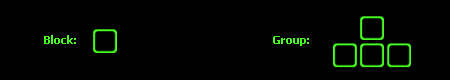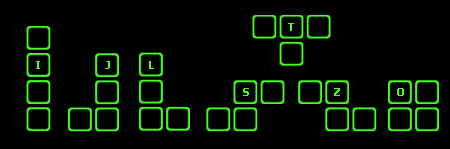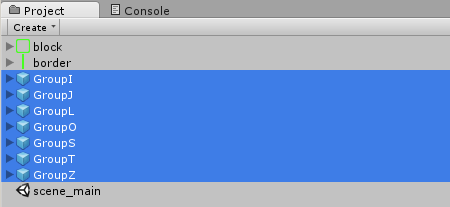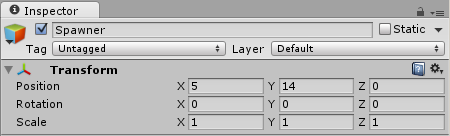# 【Unity3D开发小游戏】俄罗斯方块

@[TOC]

## 一、前言

《俄罗斯方块》（Tetris， 俄文：Тетрис）是一款由俄罗斯人阿列克谢·帕基特诺夫于1984年6月发明的休闲游戏。 该游戏曾经被多家公司代理过。经过多轮诉讼后，该游戏的代理权最终被任天堂获得。 任天堂对于俄罗斯方块来说意义重大，因为将它与GB搭配在一起后，获得了巨大的成功。 《俄罗斯方块》的基本规则是移动、旋转和摆放游戏自动输出的各种方块，使之排列成完整的一行或多行并且消除得分。 那么怎么用Unity开发《俄罗斯方块呢》，接下来跟随作者一起来看看吧。

## 二、下载链接

UI资源和源代码请搜索QQ群：1040082875下载

## 三、正文

### 关于方块和方块组我们都知道，在俄罗斯方块中有很多方块组，在教程中，我们分别给它们取名为 I, J, L, O, S, T, Z：### 创建方块组下面是各个方块的坐标：

• X=0 Y=0

• X=0 Y=1

• X=1 Y=0

• X=1 Y=1### Spawn 类``````using UnityEngine;
using System.Collections;

public class Spawner : MonoBehaviour {

// Groups
public GameObject[] groups;
}
``````

``````public void spawnNext() {
// Random Index
int i = Random.Range(0, groups.Length);

// Spawn Group at current Position
Instantiate(groups[i],
transform.position,
Quaternion.identity);
}
``````

``````void Start() {
// Spawn initial Group
spawnNext();
}
``````

### Gird 类

• 检查所有的块是否在边界内

• 检查所有的块是否都在y=0之上

• 检查一个组是否能移动到某一个位置

• 检查一行是否填满了块

• 删除一行

• 减少一行的Y坐标

 0 1 2 … 0 o x x … 1 o x o … 2 x x o … … … … … …

``````// Is there a block at (3,4)?
if (grid[3,4] != null)
// Do Stuff...
``````

``````using UnityEngine;
using System.Collections;

public class Grid : MonoBehaviour {
// The Grid itself
public static int w = 10;
public static int h = 20;
public static Transform[,] grid = new Transform[w, h];
}
``````

### roundVec2()函数

``````public static Vector2 roundVec2(Vector2 v) {
return new Vector2(Mathf.Round(v.x),
Mathf.Round(v.y));
}
``````

### insideBorder()函数

``````public static bool insideBorder(Vector2 pos) {
return ((int)pos.x >= 0 &&
(int)pos.x < w &&
(int)pos.y >= 0);
}
``````

### deleteRow()函数

``````public static void deleteRow(int y) {
for (int x = 0; x < w; ++x) {
Destroy(grid[x, y].gameObject);
grid[x, y] = null;
}
}
``````

### decreaseRow()函数

``````public static void decreaseRow(int y) {
for (int x = 0; x < w; ++x) {
if (grid[x, y] != null) {
// Move one towards bottom
grid[x, y-1] = grid[x, y];
grid[x, y] = null;

// Update Block position
grid[x, y-1].position += new Vector3(0, -1, 0);
}
}
}
``````

``````grid[x, y-1] = grid[x,y];

grid[x, y] = null;
``````

``````grid[x, y-1].position += new Vector3(0, -1, 0);
``````

### decreaseRowsAbove()函数

``````public static void decreaseRowsAbove(int y) {
for (int i = y; i < h; ++i)
decreaseRow(i);
}
``````

### isRowFull()函数

``````public static bool isRowFull(int y) {
for (int x = 0; x < w; ++x)
if (grid[x, y] == null)
return false;
return true;
}
``````

### deleteFullRows()函数

``````public static void deleteFullRows() {
for (int y = 0; y < h; ++y) {
if (isRowFull(y)) {
deleteRow(y);
decreaseRowsAbove(y+1);
--y;
}
}
}
``````

### Group脚本

``````using UnityEngine;
using System.Collections;

public class Group : MonoBehaviour {

// Use this for initialization
void Start () {

}

// Update is called once per frame
void Update () {

}
}
``````

### 创建一些函数

``````bool isValidGridPos() {
foreach (Transform child in transform) {
Vector2 v = Grid.roundVec2(child.position);

// Not inside Border?
if (!Grid.insideBorder(v))
return false;

// Block in grid cell (and not part of same group)?
if (Grid.grid[(int)v.x, (int)v.y] != null &&
Grid.grid[(int)v.x, (int)v.y].parent != transform)
return false;
}
return true;
}
``````

``````void updateGrid() {
// Remove old children from grid
for (int y = 0; y < Grid.h; ++y)
for (int x = 0; x < Grid.w; ++x)
if (Grid.grid[x, y] != null)
if (Grid.grid[x, y].parent == transform)
Grid.grid[x, y] = null;

// Add new children to grid
foreach (Transform child in transform) {
Vector2 v = Grid.roundVec2(child.position);
Grid.grid[(int)v.x, (int)v.y] = child;
}
}
``````

``````void Update() {
// Move Left
if (Input.GetKeyDown(KeyCode.LeftArrow)) {
// Modify position
transform.position += new Vector3(-1, 0, 0);

// See if valid
if (isValidGridPos())
// Its valid. Update grid.
updateGrid();
else
// Its not valid. revert.
transform.position += new Vector3(1, 0, 0);
}
}
``````

``````       1. 如果有效，更新所有子方块在grid数组的位置

2. 如果无效，向右移动
``````

``````// Move Right
else if (Input.GetKeyDown(KeyCode.RightArrow)) {
// Modify position
transform.position += new Vector3(1, 0, 0);

// See if valid
if (isValidGridPos())
// It's valid. Update grid.
updateGrid();
else
// It's not valid. revert.
transform.position += new Vector3(-1, 0, 0);
}
``````

``````// Rotate
else if (Input.GetKeyDown(KeyCode.UpArrow)) {
transform.Rotate(0, 0, -90);

// See if valid
if (isValidGridPos())
// It's valid. Update grid.
updateGrid();
else
// It's not valid. revert.
transform.Rotate(0, 0, 90);
}
``````

``````// Fall
else if (Input.GetKeyDown(KeyCode.DownArrow)) {
// Modify position
transform.position += new Vector3(0, -1, 0);

// See if valid
if (isValidGridPos()) {
// It's valid. Update grid.
updateGrid();
} else {
// It's not valid. revert.
transform.position += new Vector3(0, 1, 0);

// Clear filled horizontal lines
Grid.deleteFullRows();

// Spawn next Group
FindObjectOfType<Spawner>().spawnNext();

// Disable script
enabled = false;
}
}
``````

``````// Time since last gravity tick
float lastFall = 0;
``````

``````// Move Downwards and Fall
else if (Input.GetKeyDown(KeyCode.DownArrow) ||
Time.time - lastFall >= 1) {
// Modify position
transform.position += new Vector3(0, -1, 0);

// See if valid
if (isValidGridPos()) {
// It's valid. Update grid.
updateGrid();
} else {
// It's not valid. revert.
transform.position += new Vector3(0, 1, 0);

// Clear filled horizontal lines
Grid.deleteFullRows();

// Spawn next Group
FindObjectOfType<Spawner>().spawnNext();

// Disable script
enabled = false;
}

lastFall = Time.time;
}
``````

``````void Update() {
// Move Left
if (Input.GetKeyDown(KeyCode.LeftArrow)) {
// Modify position
transform.position += new Vector3(-1, 0, 0);

// See if valid
if (isValidGridPos())
// It's valid. Update grid.
updateGrid();
else
// It's not valid. revert.
transform.position += new Vector3(1, 0, 0);
}

// Move Right
else if (Input.GetKeyDown(KeyCode.RightArrow)) {
// Modify position
transform.position += new Vector3(1, 0, 0);

// See if valid
if (isValidGridPos())
// It's valid. Update grid.
updateGrid();
else
// It's not valid. revert.
transform.position += new Vector3(-1, 0, 0);
}

// Rotate
else if (Input.GetKeyDown(KeyCode.UpArrow)) {
transform.Rotate(0, 0, -90);

// See if valid
if (isValidGridPos())
// It's valid. Update grid.
updateGrid();
else
// It's not valid. revert.
transform.Rotate(0, 0, 90);
}

// Move Downwards and Fall
else if (Input.GetKeyDown(KeyCode.DownArrow) ||
Time.time - lastFall >= 1) {
// Modify position
transform.position += new Vector3(0, -1, 0);

// See if valid
if (isValidGridPos()) {
// It's valid. Update grid.
updateGrid();
} else {
// It's not valid. revert.
transform.position += new Vector3(0, 1, 0);

// Clear filled horizontal lines
Grid.deleteFullRows();

// Spawn next Group
FindObjectOfType<Spawner>().spawnNext();

// Disable script
enabled = false;
}

lastFall = Time.time;
}
}
``````

``````void Start() {
// Default position not valid? Then it's game over
if (!isValidGridPos()) {
Debug.Log("GAME OVER");
Destroy(gameObject);
}
}
``````